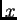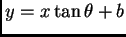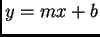MAT 331, Fall 2002
Project 1: Least-Squares Fitting
Due Wednesday, October 9

In this project, you are to fit a line to some data in three different ways. First, by using the usual'' least squares method, which minimizes the sums of the squares of the vertical distances between the data and the line. Then, find the line given by assuming that thevariable may contain the errors, and minimizing the sums of squares of the horizontal distances. Finally, you are to find the line that minimizes the sum of the squares of the absolute distances from the points to the line. (In this case, you may find it convenient to write your line asinstead of the usual; you could write the other lines that way as well; it makes no difference). See the three figures below.In the third case (minimizing the absolute Euclidean distance), the equations you need to solve to find the minimum will be nonlinear, and you will discover that there are two critical points. Only one is the minimum; what line does the other critical point correspond to? You might find it helpful to use plot3d to draw a picture of the surface that you are finding the minimum of. You should, of course, also have Maple plot the 3 lines you find along with the data points.

Your project should be written as a paper which explains clearly each step you take and why. In particular, you must give a careful derivation of the functions you minimize-- you cannot merely quote the formula for the distance from a point to a line. You may, of course, assume that the formula for the distance between two points in the plane is known to the reader. Your paper should be written so that someone who has a good working knowledge of mathematics but has never taken this class will understand what you are doing and why.

You should use Maple to do the actual computations. While it would make for a more readable paper to intersperse your Maple calculations along with your exposition, you may write your exposition first and append the Maple at the back. However, do not include false starts, mistakes, or irrelevant calculations in your finished product. Pay attention to spelling, grammar, and sentence structure. The expository part of this project counts as much as the actual calculations, and should not be ignored.

You will find a file containing the data points at

/home/mat331/www/projects/data1/username.txt,

where username is the username of your mathlab account. Use Maple's read command to load this data. Of course, this file is also available from the class web page.

MAT 331 2002-09-03